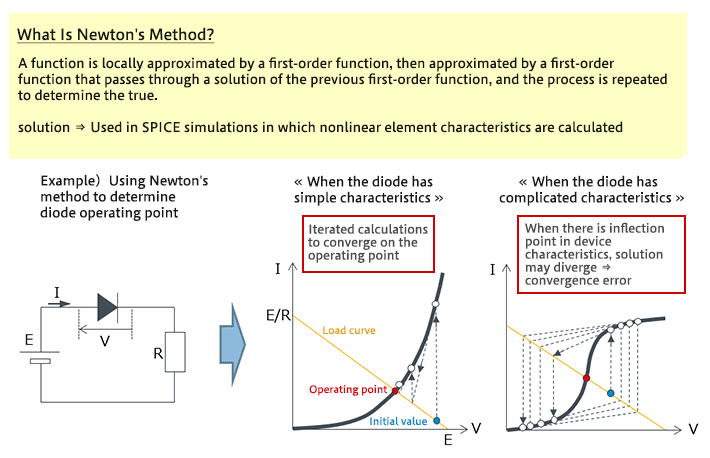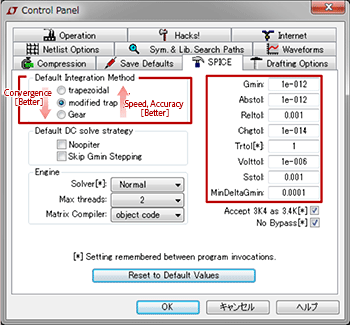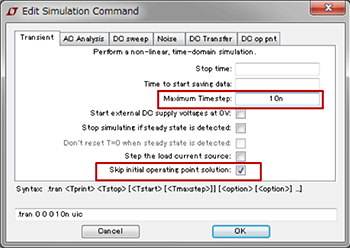# Convergence Properties and Stability of SPICE Simulations

2019.01.24

・When using a SPICE-based simulator, if there are analysis errors or unstable results, there may be problems with convergence or stability.

・In some cases, problems with convergence and stability can be avoided by changing the settings of the SPICE simulator.

・It is difficult for the user to resolve problems if the device model has defects.

In recent years, the simulation results of SPICE-based simulators have approached actual characteristics quite closely. In some cases, actual circuit characteristics are adjusted so as to come closer to simulation results as realistic yet ideal characteristics; in this and other ways, SPICE-based simulations have become quite a useful tool. However, depending on the analysis algorithm, condition settings, and device model, there may be problems with convergence properties or stability. Through an understanding of problems with simulations relating to convergence properties and stability, simulations can be utilized more effectively.

## Convergence Properties and Stability of SPICE Simulations

Problems with convergence properties in simulations include cases in which calculations do not begin even upon execution, or else stop midway, or cases in which results vary greatly for even slight changes in conditions, or cases in which the results obtained are not plausible. Put simply, these are cases of analysis errors or cases in which results are unstable.

There are a number of reasons for these cases; here we present an example that is due to the device model. Shown below is the I-V (current-voltage) characteristic of a resistor and a diode connected in series; however, simulations of a nonlinear device such as a diode are complicated compared with a linear device in which the current and the voltage are simply proportional, such as a resistor. The equation for the I-V characteristic of a resistor is something anyone can write easily, but the equation for the forward characteristic of a diode is not so familiar. In the following example, Newton’s method, which is well known for its use with SPICE simulations, is employed; in this method, approximating solutions for the operating point, which is the intersection of the load curve and the nonlinear load (in this case, the diode), are determined iteratively. When the characteristic for the device model is complex–is discontinuous, has an inflection point, or the like–results may be obtained that do not converge, or are not plausible, as in the figure on the right.## Methods of Addressing Cases of Poor Convergence or Stability

When convergence or stability properties are poor, there are a number of countermeasures available. These differ depending on the simulator, so the following should be regarded as only one example. Simulator settings include a number of items related to convergence and stability; these can be modified and the result checked. In this example, there are three algorithm options; we try changing the current selection. We also try changing convergence conditions such as those related to precision and errors.

① Try changing the algorithm to Gear

• ・trapezoidal: Fast calculations and high precision, but problematic convergence
• ・modified trap: Improved convergence compared with the trapezoidal method
• ・Gear (predictor correction method): Ease of convergence, but inferior calculation speed and precision

② Relax the convergence conditions

• ・Abstol: Current tolerance. In the case of the figure, within 1 pA.
• ・Reltol: Relative tolerance. In the case of the figure, within 0.1%.
• ・Chgtol: Electric charge tolerance. In the case of the figure, within 10 fC.
• ・Vlottol: Voltage tolerance. In the case of the figure, within 1 μV.

When the tolerance is raised (the value is lowered), calculation speed slows, but convergence properties are improved.③ Set the Maximum Timestep. The simulation can be executed even if this is left blank, but if the Timestep is too large, an error may occur.

④ If convergence is poor for the first Tran (transient analysis) DC point, setting “skip dc” is effective.The above are approaches to use in cases where convergence or stability problems can be avoided by changing simulator settings and the like on the user side. However, there are cases in which the device model has defects. In these cases, it is extremely difficult for the user to resolve simulation errors.

If there is occasion to create a device model, it is above all important that an operating device model be created emphasizing convergence and stability properties rather than precision or tolerance.